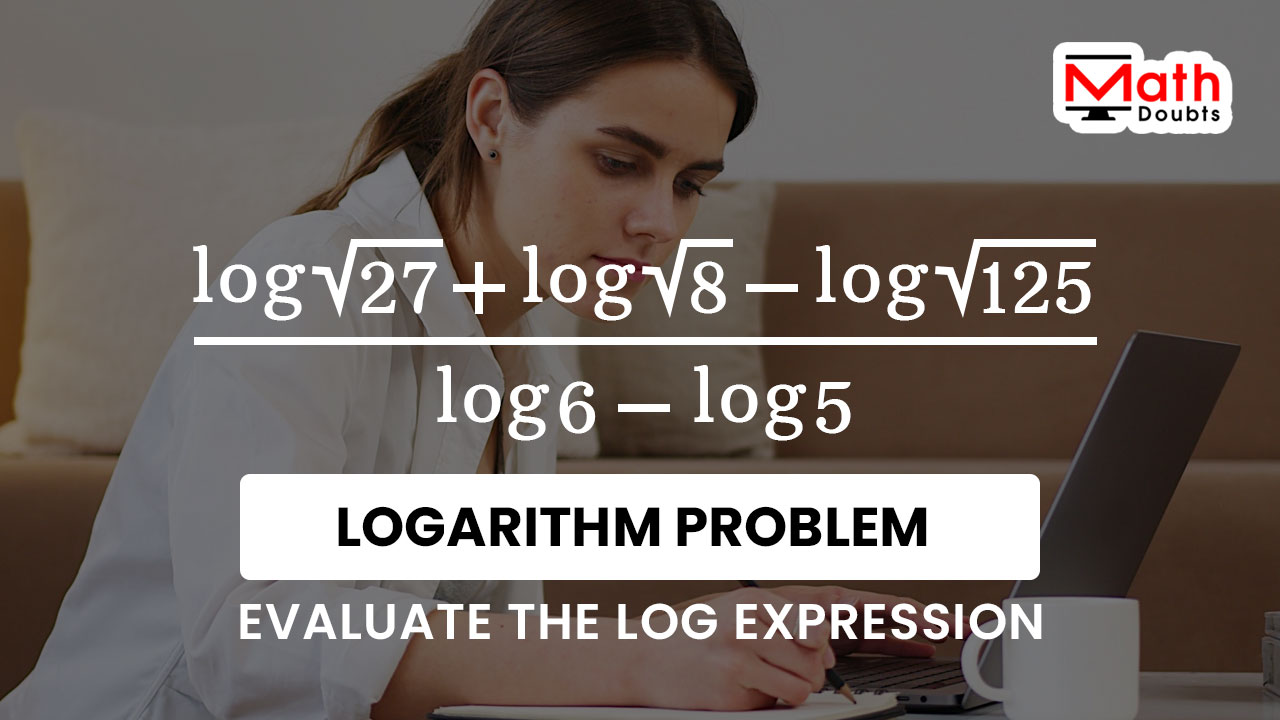# Evaluate $\dfrac{\log{\sqrt{27}}+\log{\sqrt{8}}-\log{\sqrt{125}}}{\log{6}-\log{5}}$The common logarithm of the square root of twenty seven plus logarithm of square root of eight minus logarithm of square root of one hundred twenty five divided by the logarithm of six minus logarithm of five should be evaluated in this logarithmic math problem.

### Express the quantities in exponential notation

Three numbers $27$, $8$ and $125$ are there under the square root inside the common logarithm in the numerator. Let’s first concentrate on simplifying the logarithmic expression in the numerator of the rational function. The numbers $27$, $8$ and $125$ can be split as a product of three factors.

$=\,\,$ $\dfrac{\log{\sqrt{3 \times 3 \times 3}}+\log{\sqrt{8}}-\log{\sqrt{125}}}{\log{6}-\log{5}}$

The number three is multiplied by itself twice. Now, the product of the numbers $3$ can be written in exponential notation.

$=\,\,$ $\dfrac{\log{\sqrt{3^3}}+\log{\sqrt{8}}-\log{\sqrt{125}}}{\log{6}-\log{5}}$

Similarly, use the same procedure to express the numbers $8$ and $125$ in the exponential form by the factorization.

$=\,\,$ $\dfrac{\log{\sqrt{3^3}}+\log{\sqrt{2 \times 2 \times 2}}-\log{\sqrt{125}}}{\log{6}-\log{5}}$

$=\,\,$ $\dfrac{\log{\sqrt{3^3}}+\log{\sqrt{2^3}}-\log{\sqrt{125}}}{\log{6}-\log{5}}$

$=\,\,$ $\dfrac{\log{\sqrt{3^3}}+\log{\sqrt{2^3}}-\log{\sqrt{5 \times 5 \times 5}}}{\log{6}-\log{5}}$

$=\,\,$ $\dfrac{\log{\sqrt{3^3}}+\log{\sqrt{2^3}}-\log{\sqrt{5^3}}}{\log{6}-\log{5}}$

### Eliminate the irrational form from the terms

The quantities inside the common logarithm in each term of the numerator are in irrational form. So, it is advisable to remove the irrational form from each term of logarithmic expression in the numerator. According to the arithmetic, the square root symbol can be written as an exponent $1$ divided by $2$.

$=\,\,$ $\dfrac{\log{(3^3)^{\Large \frac{1}{2}}}+\log{(2^3)^{\Large \frac{1}{2}}}-\log{(5^3)^{\Large \frac{1}{2}}}}{\log{6}-\log{5}}$

In the numerator, the quantity inside the common logarithm in each term represents the power of a quantity in exponential form but it can be simplified by the power rule of exponents.

$=\,\,$ $\dfrac{\log{\Big(3^{3 {\large \times} \Large \frac{1}{2}}\Big)}+\log{\Big(2^{3 {\large \times} \Large \frac{1}{2}}\Big)}-\log{\Big(5^{3 {\large \times} \Large \frac{1}{2}}\Big)}}{\log{6}-\log{5}}$

The number $3$ and the fraction $1$ divided by $2$ can be multiplied by the multiplication rule of the fractions.

$=\,\,$ $\dfrac{\log{\Big(3^{\Large \frac{3 \times 1}{2}}\Big)}+\log{\Big(2^{\Large \frac{3 \times 1}{2}}\Big)}-\log{\Big(5^{\Large \frac{3 \times 1}{2}}\Big)}}{\log{6}-\log{5}}$

$=\,\,$ $\dfrac{\log{\Big(3^{\Large \frac{3}{2}}\Big)}+\log{\Big(2^{\Large \frac{3}{2}}\Big)}-\log{\Big(5^{\Large \frac{3}{2}}\Big)}}{\log{6}-\log{5}}$

The numbers $3$, $2$ and $5$ consists of the fraction $3$ divided by $2$ as an exponent inside the common logarithm in every term of the numerator. Every term expresses the logarithm of a quantity in exponential notation and it can be simplified by the fundamental power rule of logarithms.

$=\,\,$ $\dfrac{\dfrac{3}{2} \times \log{3}+\dfrac{3}{2} \times \log{2}-\dfrac{3}{2} \times \log{5}}{\log{6}-\log{5}}$

### Evaluate the Rational function by simplification

The improper fraction $3$ divided by $2$ is commonly appearing as a factor in each term of the numerator. So, the fraction $3$ divided by $2$ can be taken out common from three logarithmic terms in the numerator of the rational expression.

$=\,\,$ $\dfrac{\dfrac{3}{2} \times (\log{3}+\log{2}-\log{5})}{\log{6}-\log{5}}$

The rational expression can be split as a product of two different fractions as per the multiplication rule of the fractions.

$=\,\,$ $\dfrac{3}{2} \times \dfrac{\log{3}+\log{2}-\log{5}}{\log{6}-\log{5}}$

The first factor is a rational number and the second factor is a rational expression. Now, let’s concentrate on second factor. The common logarithm of $5$ is there in both numerator and denominator. The logarithm of $6$ is already in simplified form. So, add the logarithms of $3$ and $2$ to find their sum and it can be done by the sum rule of the logarithms.

$=\,\,$ $\dfrac{3}{2} \times \dfrac{\log{(3 \times 2)}-\log{5}}{\log{6}-\log{5}}$

$=\,\,$ $\dfrac{3}{2} \times \dfrac{\log{(6)}-\log{5}}{\log{6}-\log{5}}$

$=\,\,$ $\dfrac{3}{2} \times \dfrac{\log{6}-\log{5}}{\log{6}-\log{5}}$

The common logarithm of $6$ minus logarithm of $5$ is an expression in both numerator and denominator of the rational expression. So, the quotient of the rational expression is one due to the cancellation.

$=\,\,$ $\dfrac{3}{2} \times \dfrac{\cancel{\log{6}-\log{5}}}{\cancel{\log{6}-\log{5}}}$

$=\,\,$ $\dfrac{3}{2} \times 1$

Now, it is time to multiply the fraction $3$ divided by $2$, by the number $1$ to find their product of them mathematically.

$=\,\,$ $\dfrac{3}{2}$

Latest Math Topics
Jun 26, 2023
Jun 23, 2023

###### Math Questions

The math problems with solutions to learn how to solve a problem.

Learn solutions

Practice now

###### Math Videos

The math videos tutorials with visual graphics to learn every concept.

Watch now

###### Subscribe us

Get the latest math updates from the Math Doubts by subscribing us.